# The MODEL Procedure

### SOLVE Statement

Subsections:
• SOLVE variables <SATISFY= equations> </options>;

The SOLVE statement specifies that the model be simulated or forecast for input data values and, optionally, selects the variables to be solved. If the list of variables is omitted, all of the model variables declared ENDOGENOUS are solved. If no model variables are declared ENDOGENOUS, then all model variables are solved.

The following specification can be used in the SOLVE statement:

SATISFY=equation
SATISFY=( equations )

specifies a subset of the model equations that the solution values are to satisfy. If the SATISFY= option is not used, the solution is computed to satisfy all the model equations. Note that the number of equations must equal the number of variables solved.

#### Data Set Options

DATA=SAS-data-set

names the input data set. The model is solved for each observation read from the DATA= data set. If the DATA= option is not specified on the SOLVE statement, the data set specified by the DATA= option in the PROC MODEL statement is used.

ESTDATA=SAS-data-set

names a data set whose first observation provides values for some or all of the parameters and whose additional observations (if any) give the covariance matrix of the parameter estimates. The covariance matrix read from the ESTDATA= data set is used to generate multivariate normal pseudo-random shocks to the model parameters when the RANDOM= option requests Monte Carlo simulation.

OUT=SAS-data-set

outputs the predicted (solution) values, residual values, actual values, or equation errors from the solution to a data set. The residual values are the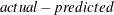values, which is the negative of RESID.variable as defined in the section Equation Translations. Only the solution values are output by default.

OUTACTUAL

outputs the actual values of the solved variables read from the input data set to the OUT= data set. This option is applicable only if the OUT= option is specified.

OUTALL

specifies the OUTACTUAL, OUTERRORS, OUTLAGS, OUTPREDICT, and OUTRESID options.

OUTERRORS

writes the equation errors to the OUT= data set. These values are normally very close to 0 when a simultaneous solution is computed; they can be used to double-check the accuracy of the solution process. This option applies only if the OUT= option is specified.

OUTLAGS

writes the observations that are used to start the lags to the OUT= data set. This option applies only if the OUT= option is specified.

OUTOBJVALS

writes the objective function value to the OBJVALS variable in the OUT= data set. The objective function value is computed only when the OPTIMIZE solution method is specified. This value is close to 0 when an unbounded simultaneous solution is computed and can be greater than 0 when bounds are active in the solution. This option applies only if the OUT= option is specified.

OUTPREDICT

writes the solution values to the OUT= data set. This option applies only if the OUT= option is specified.

The OUTPREDICT option is the default unless one of the other output options is specified.

OUTRESID

writes the residual values that are computed as thevalues and is not the same as the RESID.variable values. This option applies only if the OUT= option is specified.

OUTVIOLATIONS

writes the equation violations to the OUT= data set. The equation violations are computed only when the OPTIMIZE solution method is specified. The violations provide information about how much each equation contributes to the objective function value when bounds are active in the solution. This option applies only if the OUT= option is specified.

PARMSDATA=SAS-data-set

specifies a data set that contains the parameter estimates. See the section Input Data Sets for more details.

RESIDDATA=SAS-data-set

specifies a data set that contains the residuals to be used in the empirical distribution. This data set can be created using the OUT= option in the FIT statement.

SDATA=SAS-data-set

specifies a data set that provides the covariance matrix of the equation errors. The covariance matrix that is read from the SDATA= data set is used to generate multivariate normal pseudo-random shocks to the equations when the RANDOM= option requests Monte Carlo simulation.

TIME=name

specifies the name of the time variable. This variable must be in the data set.

TYPE=name

specifies the estimation type. The name that is specified in the TYPE= option is compared to the _TYPE_ variable in the ESTDATA= and SDATA= data sets to select observations to use in constructing the covariance matrices. When TYPE= is omitted, the last estimation type in the data set is used.

#### Solution Mode Options: Lag Processing

DYNAMIC

specifies a dynamic solution. In the dynamic solution mode, solved values are used by the lagging functions. DYNAMIC is the default.

NAHEAD=n

specifies a simulation of n-period-ahead dynamic forecasting. The NAHEAD= option is used to simulate the process of using the model to produce successive forecasts to a fixed forecast horizon, in which each forecast uses the historical data available at the time the forecast is made.

Note that NAHEAD=1 produces a static (one-step-ahead) solution. NAHEAD=2 produces a solution that uses one-step-ahead solutions for the first lag (LAG1 functions return static predicted values) and actual values for longer lags. NAHEAD=3 produces a solution that uses NAHEAD=2 solutions for the first lags, NAHEAD=1 solutions for the second lags, and actual values for longer lags. In general, NAHEAD=n solutions use NAHEAD=n–1 solutions for LAG1, NAHEAD=n–2 solutions for LAG2, and so forth.

START=s

specifies static solutions until the sth observation and then changes to dynamic solutions. If the START=s option is specified, the first observation in the range in which LAGn delivers solved predicted values is s+n, while LAGn returns actual values for earlier observations.

STATIC

specifies a static solution. In static solution mode, actual values of the solved variables from the input data set are used by the lagging functions.

#### Solution Mode Options: Use of Available Data

FORECAST

specifies that the actual value of a solved variable is used as the solution value (instead of the predicted value from the model equations) whenever nonmissing data are available in the input data set. That is, in FORECAST mode, PROC MODEL solves only for those variables that are missing in the input data set.

SIMULATE

specifies that PROC MODEL always solves for all solution variables as a function of the input values of the other variables, even when actual data for some of the solution variables are available in the input data set. SIMULATE is the default.

#### Solution Mode Options: Numerical Solution Method

JACOBI

computes a simultaneous solution using a Jacobi iteration.

NEWTON

computes a simultaneous solution by using Newton’s method. When the NEWTON option is selected, the analytic derivatives of the equation errors with respect to the solution variables are computed, and memory-efficient sparse matrix techniques are used for factoring the Jacobian matrix.

The NEWTON option can be used to solve both normalized-form and general-form equations and can compute goal-seeking solutions. NEWTON is the default.

OPTIMIZE

computes a simultaneous solution by minimizing a norm of the equation errors with respect to the solution variables. The OPTIMIZE method obeys constraints on the solution variables that are imposed by the BOUNDS and RESTRICT statements.

SEIDEL

computes a simultaneous solution by using a Gauss-Seidel method.

SINGLE
ONEPASS

specifies a single-equation (nonsimultaneous) solution. The model is executed once to compute predicted values for the variables from the actual values of the other endogenous variables. The SINGLE option can be used only for normalized-form equations and cannot be used for goal-seeking solutions.

For more information about these options, see the section Solution Modes.

#### Monte Carlo Simulation Options

COPULA=(copula-options)

specifies the copula to be used in the simulation. You can specify the following copula-options:

• CLAYTON(), whereis the Clayton copula parameter

• FRANK(), whereis the Frank copula parameter

• GUMBEL(), whereis the Gumbel copula parameter

• NORMAL

• NORMALMIX( n,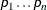,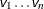), where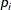are the probabilities and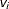are the variances

• T(df) <ASYM>, where df is the degrees-of-freedom parameter

The normal (Gaussian) copula is the default. The copula applies to covariance of equation errors.

PSEUDO=DEFAULT | TWISTER

specifies which pseudo-number generator to use in generating draws for Monte Carlo simulation. The two pseudo-random number generators that are supported by the MODEL procedure are a default congruential generator that has period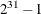and a Mersenne-Twister pseudo-random number generator that has an extraordinarily long period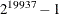.

QUASI=NONE|SOBOL|FAURE

specifies a pseudo- or quasi-random number generator. Two quasi-random number generators are supported by the MODEL procedure: the Sobol sequence (QUASI=SOBOL) and the Faure sequence (QUASI=FAURE). The default is QUASI=NONE, which is the pseudo-random number generator.

RANDOM=n

repeats the solution n times for each BY group, with different random perturbations of the equation errors if the SDATA= option is specified; with different random perturbations of the parameters if the ESTDATA= option is specified and the ESTDATA= data set contains a parameter covariance matrix; and with different values returned from the random number generator functions, if any are used in the model program. If RANDOM=0, the random number generator functions always return zero. See the section Monte Carlo Simulation for details. The default is RANDOM=0.

SEED=n

specifies an integer to use as the seed in generating pseudo-random numbers to shock the parameters and equations when the ESTDATA= or SDATA= option is specified. If n is negative or 0, the time of day from the computer’s clock is used as the seed. The SEED= option is relevant only if the RANDOM= option is specified. The default is SEED=0.

WISHART=df

specifies that a Wishart distribution with degrees of freedom df be used in place of the normal error covariance matrix. This option is used to model the variance of the error covariance matrix when Monte Carlo simulation is selected.

#### Options for Controlling the Numerical Solution Process

The following options are useful when you have difficulty converging to the simultaneous solution.

CONVERGE=value

specifies the convergence criterion for the simultaneous solution. Convergence of the solution is judged by comparing the CONVERGE= value to the maximum over the equations of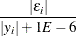if they are computable, otherwise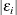whererepresents the equation error and yrepresents the solution variable that corresponds to the ith equation for normalized-form equations. The default is CONVERGE=1E–8.

MAXITER=n

specifies the maximum number of iterations allowed for computing the simultaneous solution for any observation. The default is MAXITER=50.

MAXSUBITER=n

specifies the maximum number of damping subiterations that are performed in solving a nonlinear system when using the NEWTON solution method. Damping is disabled by setting MAXSUBITER=0. The default is MAXSUBITER=10.

#### Printing Options

INTGPRINT

prints between data points integration values for the DERT. variables and the auxiliary variables. If you specify the DETAILS option, the integrated derivative variables are printed as well.

ITPRINT

prints the solution approximation and equation errors at each iteration for each observation. This option can produce voluminous output.

PRINTALL

specifies the printing control options DETAILS, ITPRINT, SOLVEPRINT, STATS, and THEIL.

SOLVEPRINT

prints the solution values and residuals at each observation.

STATS

prints various summary statistics for the solution values.

THEIL

prints tables of Theil inequality coefficients and Theil relative change forecast error measures for the solution values. See the section Summary Statistics for more information.

#### Other Options

Other options that can be used on the SOLVE statement include the following that list and analyze the model: BLOCK, GRAPH, LIST, LISTCODE, LISTDEP, LISTDER, and XREF. The LTEBOUND= and MINTIMESTEP= options can be used to control the integration process. The following printing-control options are also available: DETAILS, FLOW, MAXERRORS=, NOPRINT, and TRACE. For complete descriptions of these options, see the PROC MODEL and FIT statement options described earlier in this chapter.